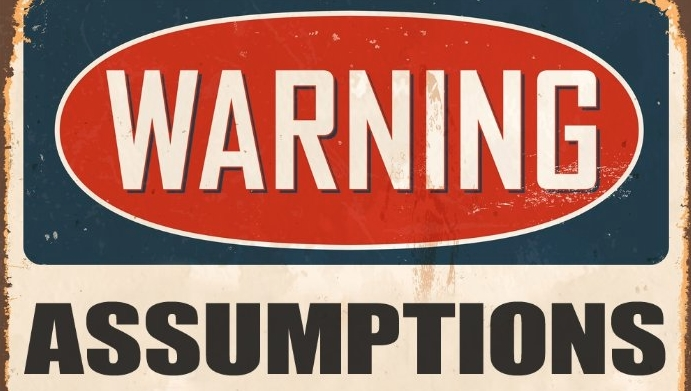# How to solve assumption based CAT RC and CR Questions

CAT 2020 MOCK Test Series at INR 1000/- only.

## Assumptions:

The Critical Reasoning section typically includes several questions that test your ability to identify assumptions of arguments. An assumption of an argument plays a role in establishing the conclusion. However, unlike a premise, an assumption is not something that the arguer explicitly asserts to be true; an assumption is instead just treated as true for the purposes of the argument.

Although assumptions can be stated explicitly in an argument, Critical Reasoning questions that ask about assumptions ask only about unstated assumptions. Unstated (or tacit) assumptions can figure only in arguments that are not entirely complete, that is, in arguments in which some of the things required to establish the conclusion are left unstated. There is thus at least one significant gap in such an argument.

Assumptions relate to the gaps in an argument in two different ways. An assumption is a sufficient one if adding it to the argument’s premises would produce a conclusive argument, that is, an argument with no gaps in its support for the conclusion. An assumption is a necessary one if it is something that must be true in order for the argument to succeed.

Typical wordings of questions that ask you to identify sufficient assumptions are:

Which one of the following, if assumed, enables the conclusion of the argument to be properly drawn?

The conclusion follows logically from the premises if which one of the following is assumed?

An Example:

John comes to college in a Mercedes. He, therefore, must be rich.

The conclusion follows logically if which one of the following is assumed?

In order to approach this question, you first have to identify the conclusion of the argument and the premises offered in its support. In this case, the conclusion is signaled by the conclusion indicator “therefore” and reads “…He (John) must be rich.” There is only one consideration explicitly presented in support of this conclusion: John comes to college in a Mercedes. Note that the premise talks only about John’s coming to college in a Mercedes. It makes no reference about John being rich. Thus there is a gap between what is given to us as a premise, and what has been concluded on the basis of that premise. For the conclusion to follow logically, this gap has to be bridged. The assumption is this gap; in other words, the assumption is the unstated premise that helps us further support the author’s conclusion

John comes to college in a Mercedes. John, therefore, must be rich.

Assumpti0n: Mercedes can be owned only by rich people

## How to approach assumption questions?

Negation of the assumption is one of the most common methods to arrive at the right answer choice. In other words, if your assumption is correct, negating it will weaken your conclusion

In the example above, let’s negate the assumption. The negated statement will be: Mercedes need not be necessarily owned by rich people.

If this statement is true, then John need not necessarily be rich because even non-rich people can own a Mercedes. Thus the conclusion is weakened.